Browse by Topic
Related Topics

# The Ease of Wolfram|Alpha, the Power of Mathematica: Introducing Wolfram|Alpha Notebook Edition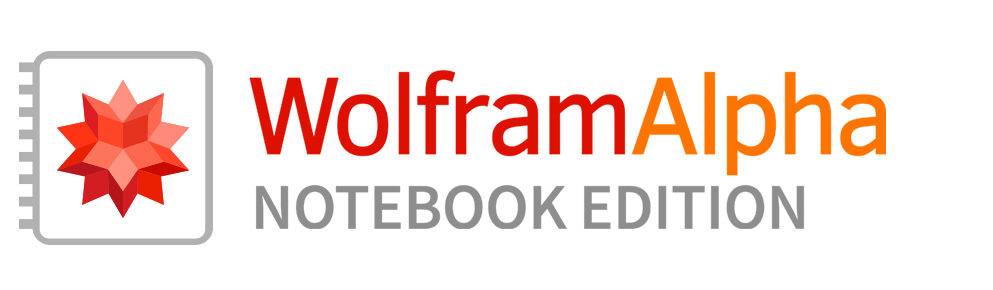## The Next Big Step for Wolfram|Alpha

Wolfram|Alpha has been a huge hit with students. Whether in college or high school, Wolfram|Alpha has become a ubiquitous way for students to get answers. But it’s a one-shot process: a student enters the question they want to ask (say in math) and Wolfram|Alpha gives them the (usually richly contextualized) answer. It’s incredibly useful—especially when coupled with its step-by-step solution capabilities.

But what if one doesn’t want just a one-shot answer? What if one wants to build up (or work through) a whole computation? Well, that’s what we created Mathematica and its whole notebook interface to do. And for more than 30 years that’s how countless inventions and discoveries have been made around the world. It’s also how generations of higher-level students have been taught.

But what about students who aren’t ready to use Mathematica yet? What if we could take the power of Mathematica (and what’s now the Wolfram Language), but combine it with the ease of Wolfram|Alpha?

Well, that’s what we’ve done in Wolfram|Alpha Notebook Edition.

It’s built on a huge tower of technology, but what it does is to let any student—without learning any syntax or reading any documentation—immediately build up or work through computations. Just type input the way you would in Wolfram|Alpha. But now you’re not just getting a one-shot answer. Instead, everything is in a Wolfram Notebook, where you can save and use previous results, and build up or work through a whole computation: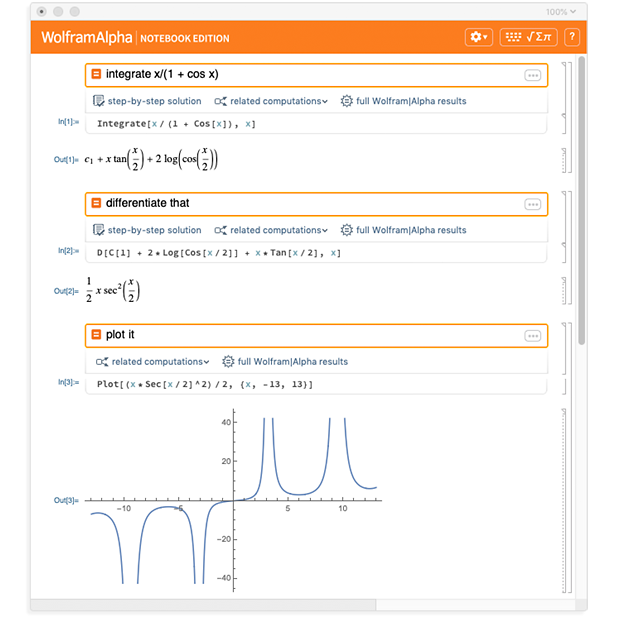## The Power of Notebooks

Being able to use Wolfram|Alpha-style free-form input is what opens Wolfram|Alpha Notebook Edition up to the full range of students. But it’s the use of the notebook environment that makes it so uniquely valuable for education. Because by being able to work through things in a sequence of steps, students get to really engage with the computations they’re doing.

Try one step. See what happens. Change it if you want. Understand the output. See how it fits into the next step. And then—right there in the notebook—see how all your steps fit together to give your final results. And then save your work in the notebook, to continue—or review what you did—another time.

But notebooks aren’t just for storing computations. They can also contain text and structure. So students can use them not just to do their computations, but also to keep notes, and to explain the computations they’re doing, or the results they get: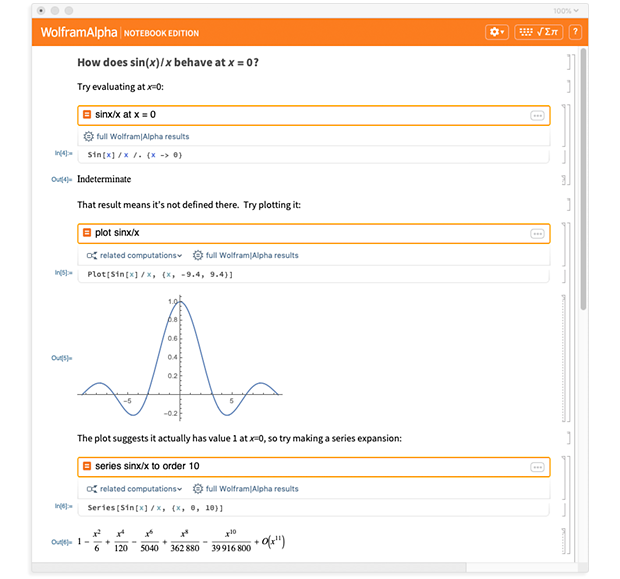And in fact, Wolfram Notebooks enable a whole new kind of student work: computational essays. A computational essay has both text and computation—combined to build up a narrative to which both human and computer contribute.

The process of creating a computational essay is a great way for students to engage with material they’re studying. Computational essays can also provide a great showcase of student achievement, as well as a means of assessing student understanding. And they’re not just something to produce for an assignment: they’re active computable documents that students can keep and use at any time in the future.

But students aren’t the only ones to produce notebooks. In Wolfram|Alpha Notebook Edition, notebooks are also a great medium for teachers to provide material to students. Describe a concept in a notebook, then let students explore by doing their own computations right there in the notebook. Or make a notebook defining an assignment or a test—then let the students fill in their work (and grade it right there in the notebook).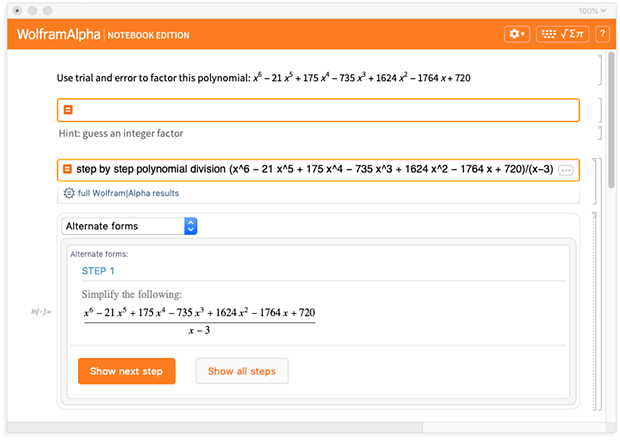It’s very common to use Wolfram|Alpha Notebook Edition to create visualizations of concepts. Often students will just ask for the visualizations themselves. But teachers can also set up templates for visualizations, and let students fill in their own functions or data to explore for themselves.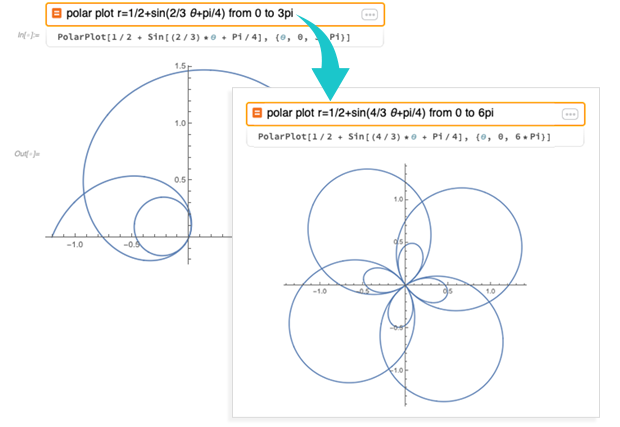Wolfram|Alpha Notebook Edition also supports dynamic interactive visualizations—for example using the Wolfram Language Manipulate function. And in Wolfram|Alpha Notebook Edition students (and teachers!) can build all sorts of dynamic visualizations just using natural language: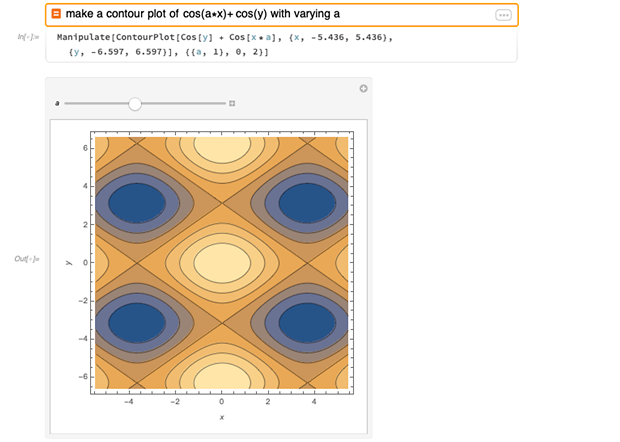But what if you want some more sophisticated interactive demonstration, that might be hard to specify? Well, Wolfram|Alpha Notebook Edition has direct access to the Wolfram Demonstrations Project, which contains over 12,000 Demonstrations. You can ask for Demonstrations using natural language, or you can just browse the Demonstrations Project website, select a Demonstration, copy it into your Wolfram|Alpha Notebook Edition notebook, and then immediately use it there: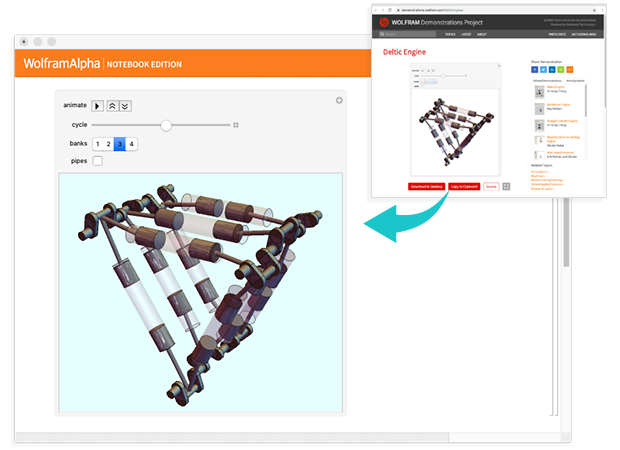With Wolfram|Alpha Notebook Edition it’s very easy to create compelling content. The content can involve pure calculations or visualizations. But—using the capabilities of the Wolfram Knowledgebase—it can also involve a vast range of real-world data, whether about countries, chemicals, words or artworks. And you can access it using natural language, and work with it directly in a notebook: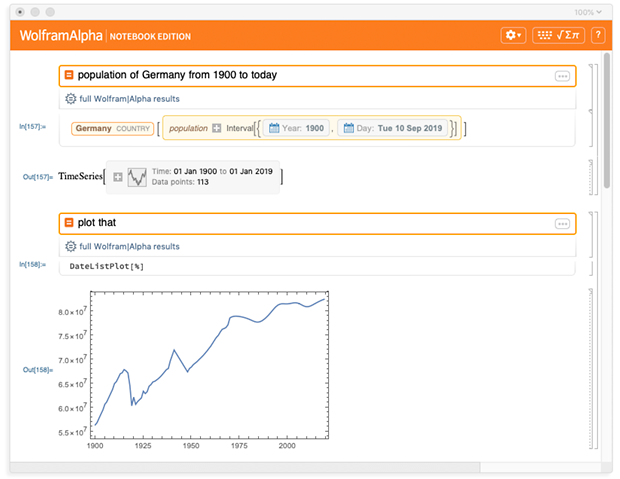Wolfram|Alpha Notebook Edition is a great tool for students to use on their own computers. But it’s also a great tool for lectures and class demonstrations (as well as for student presentations). Go to File > New > Presenter Notebook, and you’ll get a notebook that’s set up to create a Wolfram|Alpha Notebook Edition slide show: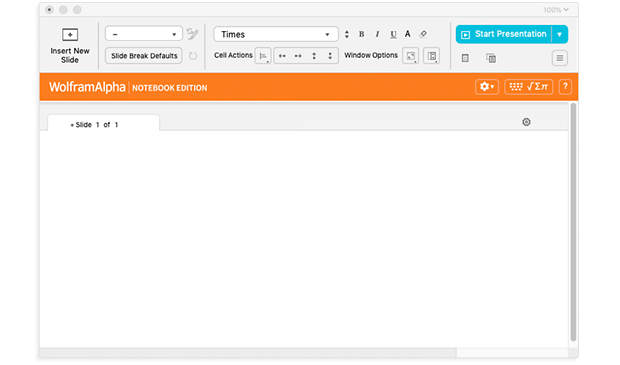Click Start Presentation and you can start presenting. But what you’ll have is not just a “PowerPoint-style” slide show. It’s a fully interactive, editable, computable slide show. The Manipulate interfaces work. Everything is immediately editable. And you can do computations right there during the presentation, exploring different cases, pulling in different data, and so on.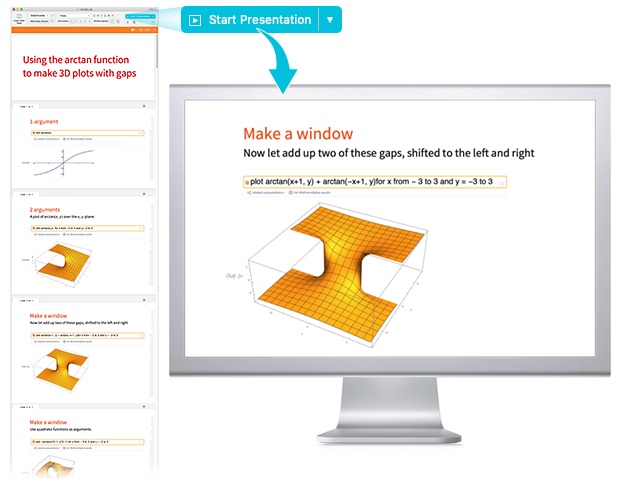## Making Code from Natural Language

We invented notebooks more than 30 years ago, and they’ve been widely used in Mathematica ever since. But while in Mathematica (and Wolfram Desktop) notebooks you (by default) specify computations in the precise syntax and semantics of the Wolfram Language, in Wolfram|Alpha Notebook Edition notebooks you instead specify them just using free-form Wolfram|Alpha-style input.

And indeed one of the key technical achievements that’s made Wolfram|Alpha Notebook Edition possible is that we’ve now developed increasingly robust natural-language-to-code technology that’s able to go from the free-form natural language input you type to precise Wolfram Language code that can be used to build up computations: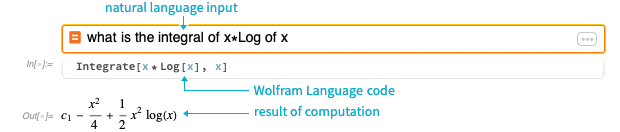By default, Wolfram|Alpha Notebook Edition is set up to show you the Wolfram Language code it generates. You don’t need to look at this code (and you can set it to always be hidden). But—satisfyingly for me as a language designer—students seem to find it very easy to read, often actually easier than math. And reading it gives them an extra opportunity to understand what’s going on—and to make sure the computation they’ve specified is actually the one they want.

And there’s a great side effect to the fact that Wolfram|Alpha Notebook Edition generates code: through routinely being exposed to code that represents natural language they’ve entered, students gradually absorb the idea of expressing things in computational language, and the concepts of computational thinking.

If a student wants to change a computation when they’re using Wolfram|Alpha Notebook Edition, they can always edit the free-form input they gave. But they can also directly edit the Wolfram Language that’s been generated, giving them real computational language experience.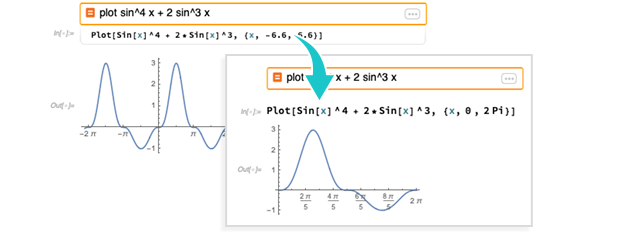## What Should I Do Next? The Predictive Interface

A central goal of Wolfram|Alpha Notebook Edition is to be completely “self-service”—so that students at all levels can successfully use it without any outside instruction or assistance. Of course, free-form input is a key part of achieving this. But another part is the Wolfram|Alpha Notebook Edition Predictive Interface—that suggests what to do next based on what students have done.

Enter a computation and you’ll typically see some buttons pop up under the input field: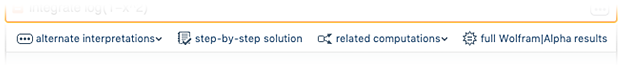These buttons will suggest directions to take. Here step-by-step solution generates an enhanced interactive version of Wolfram|Alpha Pro step-by-step functionality—all right in the notebook: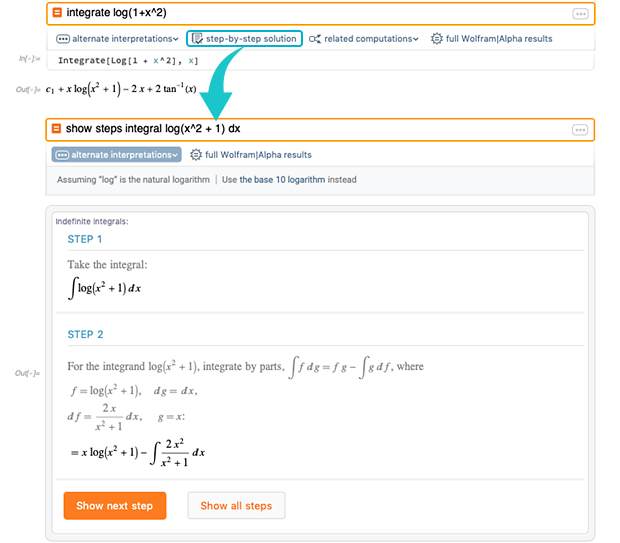Click related computations and you’ll see suggestions for different computations you might want to do: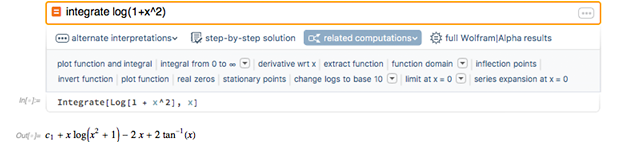It suggests plotting the integrand and the integral: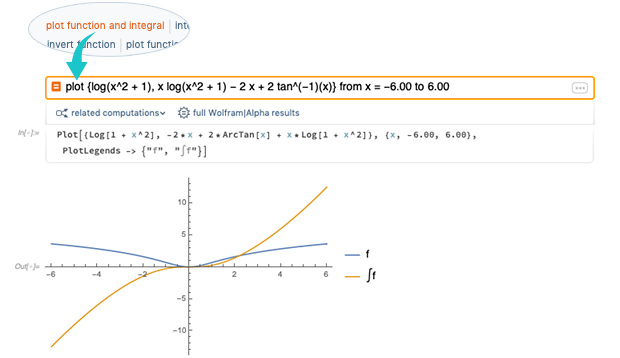It also suggests you might like to see a series expansion: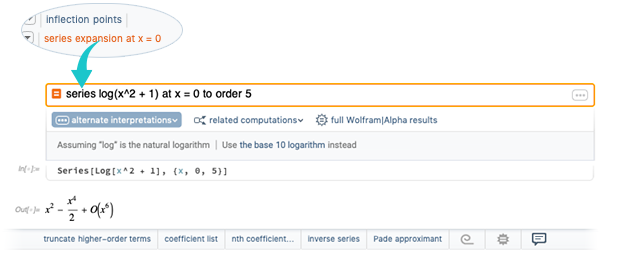Now notice that underneath the output there’s a bar of suggestions about possible follow-on computations to do on this output. Click, for example, coefficient list to find the list of coefficients: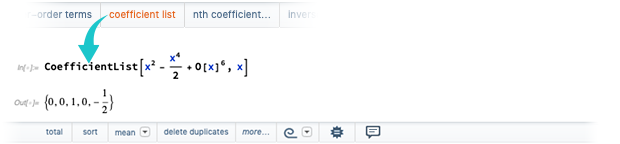Now there are new suggestions. Click, for example, total to find the total of the coefficients: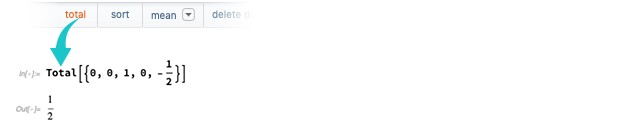## The Math Experience

Wolfram|Alpha Notebook Edition has got lots of features to enhance the “math experience”. For example, click the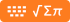button at the top of the notebook and you’ll get a “math keyboard” that you can use to directly enter math notation: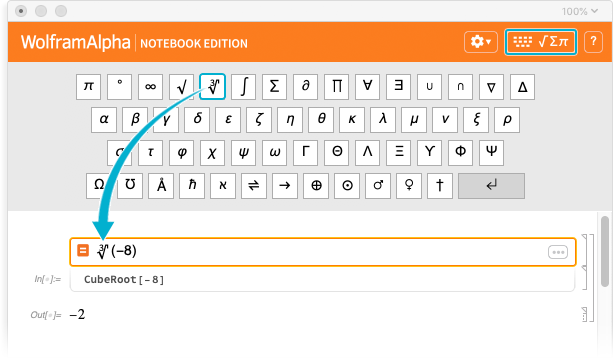The Wolfram Language that underlies Wolfram|Alpha Notebook Edition routinely handles the math that’s needed by the world’s top mathematicians. But having all that sophisticated math can sometimes lead to confusions for students. So in Wolfram|Alpha Notebook Edition there are ways to say “keep the math simple”. For example, you can set it to minimize the use of complex numbers: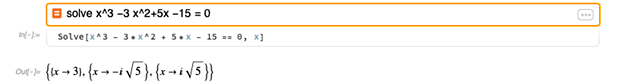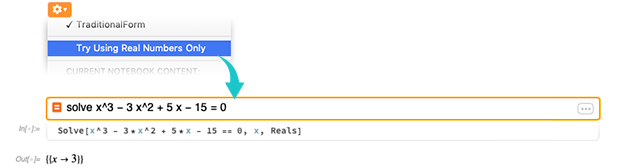Wolfram|Alpha Notebook Edition also by default does things like adding constants of integration to indefinite integrals: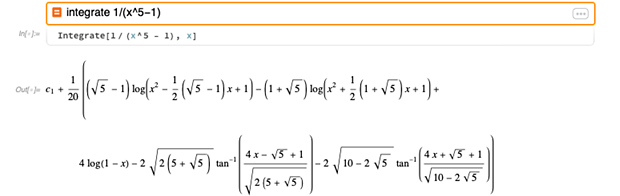By the way, Wolfram|Alpha Notebook Edition by default automatically formats mathematical output in elegant “traditional textbook” form. But it always includes a little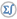button next to each output, so you can toggle between “traditional form”, and standard Wolfram Language form.

It’s quite common in doing math to have a function, and just say “I want to plot that!” But what range should you use? In Mathematica (or the Wolfram Language), you’d have to specify it. But in Wolfram|Alpha Notebook Edition there’s always an automatic range that’s picked: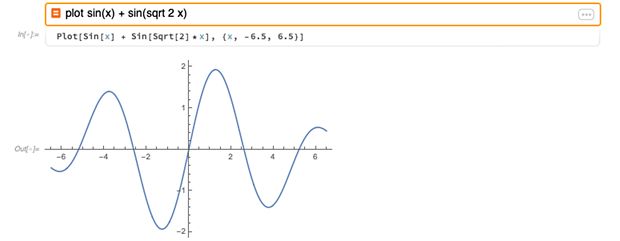But since you can see the Wolfram Language code—including the range—it’s easy to change that, and specify whatever range you want.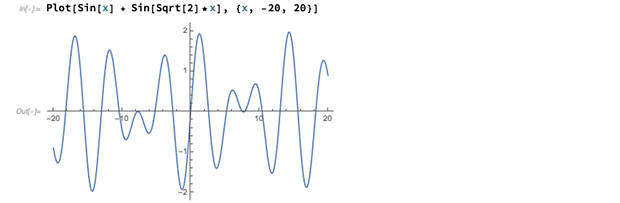What if you want to get an interactive control to change the range, or to change a parameter in the function? In Mathematica (or the Wolfram Language) you’d have to write a Manipulate. But in Wolfram|Alpha Notebook Edition, you can build a whole interactive interface just using natural language: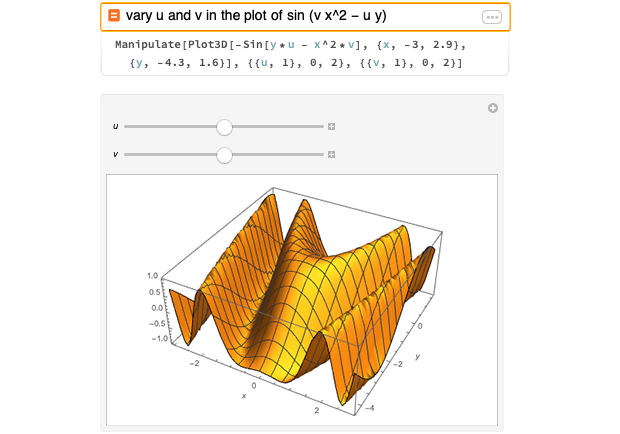And because in Wolfram|Alpha Notebook Edition the Manipulate computations are all running directly on your local computer, nothing is being slowed down by network transmission—and so everything moves at full speed. (Also, if you have a long computation, you can just let it keep running on your computer; there’s no timeout like in Wolfram|Alpha on the web.)

## Multistep Computation

One of the important features of Wolfram|Alpha Notebook Edition is that it doesn’t just do one-shot computations; it allows you to do multistep computations that in effect involve a back-and-forth conversation with the computer, in which you routinely refer to previous results: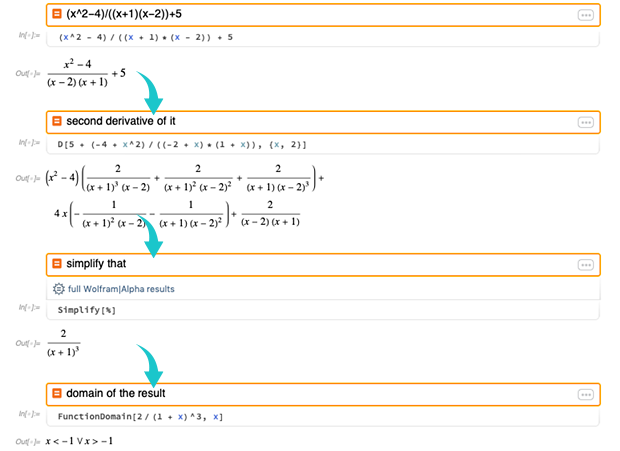Often it’s enough to just talk about the most recent result, and say things like “plot it as a function x”. But it’s also quite common to want to refer back to results earlier in the notebook. One way to do this is to say things like “the result before last”—or to use the Out[n] labels for each result. But another thing that Wolfram|Alpha Notebook Edition allows you to do is to set values of variables, that you can then use throughout your session: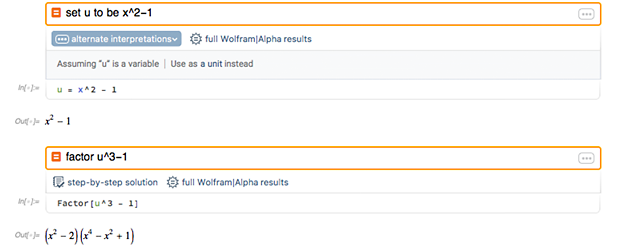It’s also possible to define functions, all with natural language: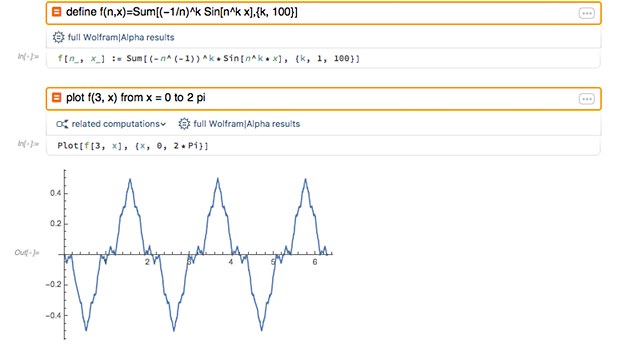There are lots of complicated design and implementation issues that arise in dealing with multistep computations. For example, if you have a traditional result for an indefinite integral, with a constant of integration, what do you do with the constant when you want to plot the result? (Wolfram|Alpha Notebook Edition consistently handles arbitrary additive constants in plots by effectively setting them to zero.)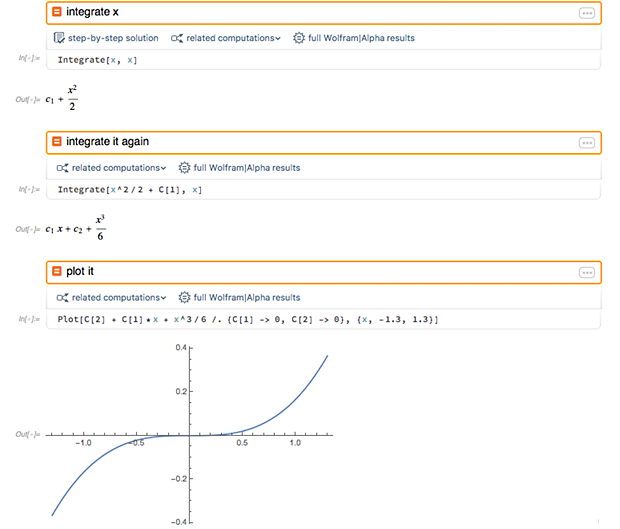It can also be complicated to know what refers to what in the “conversation”. If you say “plot”, are you trying to plot your latest result, or are you asking for an interface to create a completely new plot? If you use a pronoun, as in “plot it”, then it’s potentially more obvious what you mean, and Wolfram|Alpha Notebook Edition has a better chance of being able to use its natural language understanding capabilities to figure it out.

## The World with Wolfram|Alpha Notebook Edition

It’s been very satisfying to see how extensively Wolfram|Alpha has been adopted by students. But mostly that adoption has been outside the classroom. Now, with Wolfram|Alpha Notebook Edition, we’ve got a tool that can immediately be put to use in the classroom, across the whole college and precollege spectrum. And I’m excited to see how it can streamline coursework, deepen understanding, enable new concepts to be taught, and effectively provide a course-based personal AI tutor for every student.

Starting today, Wolfram|Alpha Notebook Edition is available on all standard computer platforms (Mac, Windows, Linux). (A cloud version will also be available on the web soon.) Colleges and universities with full Wolfram Technology System site licenses can automatically start using Wolfram|Alpha Notebook Edition today; at schools with other site licenses, it can immediately be added. It’s available to K–12 schools and junior colleges in classroom packs, or as a site license. And, of course, it’s also available to individual teachers, students, hobbyists and others.

(Oh, and if you have Mathematica or Wolfram Desktop, it’ll also be possible in future versions to create “Wolfram|Alpha mode” notebooks that effectively integrate Wolfram|Alpha Notebook Edition capabilities. And in general there’s perfect compatibility among Wolfram|Alpha Notebook Edition, Mathematica, Wolfram Desktop, Wolfram Cloud, Wolfram Programming Lab, etc.—providing a seamless experience for people progressing across education and through professional careers.)

Like Wolfram|Alpha—and the Wolfram Language—Wolfram|Alpha Notebook Edition will continue to grow in capabilities far into the future. But what’s there today is already a remarkable achievement that I think will be transformative in many educational settings.

More than 31 years ago we introduced Mathematica (and what’s now the Wolfram Language). A decade ago we introduced Wolfram|Alpha. Now, today, with the release of Wolfram|Alpha Notebook Edition we’re giving a first taste—in the context of education—of a whole new approach to computing: a full computing environment that’s driven by natural language. It doesn’t supplant Wolfram Language, or Wolfram|Alpha—but it defines a new direction that in time will bring the power of computation to a whole massive new audience.

To comment, please visit the copy of this post at Stephen Wolfram Writings »